Updating search results...

# 51 Results

View
Selected filters:
• MCCRS.Math.Content.7.RP.A.1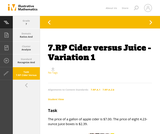Unrestricted Use
CC BY
Rating
0.0 stars

This is a task from the Illustrative Mathematics website that is one part of a complete illustration of the standard to which it is aligned. Each task has at least one solution and some commentary that addresses important aspects of the task and its potential use.

Subject:
Ratios and Proportions
Material Type:
Activity/Lab
Provider:
Illustrative Mathematics
Provider Set:
Illustrative Mathematics
Author:
Illustrative Mathematics
08/06/2015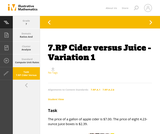Unrestricted Use
CC BY
Rating
0.0 stars

This is a task from the Illustrative Mathematics website that is one part of a complete illustration of the standard to which it is aligned. Each task has at least one solution and some commentary that addresses important aspects of the task and its potential use.

Subject:
Ratios and Proportions
Material Type:
Activity/Lab
Provider:
Illustrative Mathematics
Provider Set:
Illustrative Mathematics
Author:
Illustrative Mathematics
08/06/2015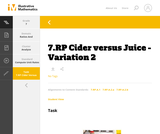Unrestricted Use
CC BY
Rating
0.0 stars

This is a task from the Illustrative Mathematics website that is one part of a complete illustration of the standard to which it is aligned. Each task has at least one solution and some commentary that addresses important aspects of the task and its potential use.

Subject:
Ratios and Proportions
Material Type:
Activity/Lab
Provider:
Illustrative Mathematics
Provider Set:
Illustrative Mathematics
Author:
Illustrative Mathematics
08/06/2015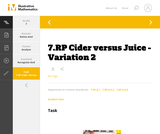Unrestricted Use
CC BY
Rating
0.0 stars

This is a task from the Illustrative Mathematics website that is one part of a complete illustration of the standard to which it is aligned. Each task has at least one solution and some commentary that addresses important aspects of the task and its potential use.

Subject:
Ratios and Proportions
Material Type:
Activity/Lab
Provider:
Illustrative Mathematics
Provider Set:
Illustrative Mathematics
Author:
Illustrative Mathematics
08/06/2015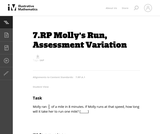Unrestricted Use
CC BY
Rating
0.0 stars

This is a task from the Illustrative Mathematics website that is one part of a complete illustration of the standard to which it is aligned. Each task has at least one solution and some commentary that addresses important asects of the task and its potential use. Here are the first few lines of the commentary for this task: Molly ran $\frac{2}{3}$ of a mile in 8 minutes. If Molly runs at that speed, how long will it take her to run one mile? [_____]...

Subject:
Mathematics
Material Type:
Activity/Lab
Provider:
Illustrative Mathematics
Provider Set:
Illustrative Mathematics
Author:
Illustrative Mathematics
03/18/2013Conditional Remix & Share Permitted
CC BY-NC
Rating
0.0 stars

In this problem-based learning module, students will be asked to brainstorm ideas and think innovatively both independently and collaboratively in addressing a real-world problem that is relevant to their daily lives and  health.  Are students aware of their calorie intake and how it affects their overall health? Students will investigate the calories consumed in a typical day and how much physical activity is needed to stay healthy and fit.  Students/teams will be encouraged to use the internet for research purposes in their design phase. Students will utilize various online platforms to design an infographic that can be shared with relevant individuals in the community and others in the school building

Subject:
Health, Medicine and Nursing
English Language Arts
Mathematics
Material Type:
Lesson Plan
Author:
Blended Learning Teacher Practice Network
07/27/2018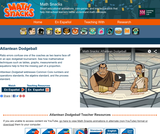Rating
0.0 stars

Ratio errors confuse a dodgeball coach as two teams face off in an epic tournament. See how mathematical techniques such as tables, graphs, measurements and equations help to find the missing part of a proportion.

Subject:
Mathematics
Material Type:
Lecture
Provider:
Learning Games Lab
Author:
NMSU Learning Gams Lab
07/15/2015Rating
0.0 stars

True love has the right ratio. In this humorous animation, the number of words spoken by each partner predicts whether a date goes well or horribly. What do you do when someone asks if you listen to country music backwards, but won't let you get a word in edgewise?

Subject:
Mathematics
Material Type:
Lecture
Provider:
Learning Games Lab
Author:
NMSU Learning Games Lab
07/15/2015Conditional Remix & Share Permitted
CC BY-NC-SA
Rating
0.0 stars

This task was developed by high school and postsecondary mathematics and agriculture sciences educators, and validated by content experts in the Common Core State Standards in mathematics and the National Career Clusters Knowledge & Skills Statements. It was developed with the purpose of demonstrating how the Common Core and CTE Knowledge & Skills Statements can be integrated into classroom learning - and to provide classroom teachers with a truly authentic task for either mathematics or CTE courses.

Subject:
Ratios and Proportions
Material Type:
Activity/Lab
Lesson Plan
Provider:
National Association of State Directors of Career Technical Education Consortium
Provider Set:
Career Technical Education
07/26/2012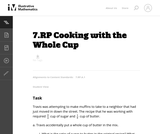Unrestricted Use
CC BY
Rating
0.0 stars

While the task as written does not explicitly use the term "unit rate," most of the work students will do amounts to finding unit rates. A recipe context works especially well since there are so many different pair-wise ratios to consider.

Subject:
Mathematics
Material Type:
Activity/Lab
Provider:
Illustrative Mathematics
Provider Set:
Illustrative Mathematics
Author:
Illustrative Mathematics
05/01/2012Only Sharing Permitted
CC BY-NC-ND
Rating
5.0 stars

This lesson unit is intended to help you assess whether students recognize relationships of direct proportion and how well they solve problems that involve proportional reasoning. In particular, it is intended to help you identify those students who: use inappropriate additive strategies in scaling problems, which have a multiplicative structure; rely on piecemeal and inefficient strategies such as doubling, halving, and decomposition, and have not developed a single multiplier strategy for solving proportionality problems; and see multiplication as making numbers bigger, and division as making numbers smaller.

Subject:
Numbers and Operations
Material Type:
Assessment
Lesson Plan
Provider:
Shell Center for Mathematical Education
Provider Set:
Mathematics Assessment Project (MAP)
04/26/2013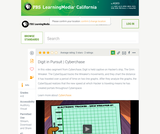Educational Use
Rating
0.0 stars

The CyberSquad tracks Digital position in time and then studies graphs to figure out what Hacker is scheming in this video from Cyberchase.

Subject:
Mathematics
Physics
Material Type:
Lecture
Provider:
PBS LearningMedia
Provider Set:
PBS Learning Media: Multimedia Resources for the Classroom and Professional Development
Author:
U.S. Department of Education
WNET
09/25/2008Only Sharing Permitted
CC BY-NC-ND
Rating
4.5 stars

This lesson unit is intended to help assess how well students are able to interpret and use scale drawings to plan a garden layout. This involves using proportional reasoning and metric units.

Subject:
Algebra
Geometry
Ratios and Proportions
Material Type:
Assessment
Lesson Plan
Provider:
Shell Center for Mathematical Education
Provider Set:
Mathematics Assessment Project (MAP)
04/26/2013Conditional Remix & Share Permitted
CC BY-NC
Rating
0.0 stars

Math in Real Life (MiRL) supports the expansion of regional networks to create an environment of innovation in math teaching and learning.  The focus on applied mathematics supports the natural interconnectedness of math to other disciplines while infusing relevance for students.  MiRL supports a limited number of networked math learning communities that focus on developing and testing applied problems in mathematics.  The networks help math teachers refine innovative teaching strategies with the guidance of regional partners and the Oregon Department of Education.

Subject:
Mathematics
Material Type:
Homework/Assignment
Author:
Mark Freed
Tom Thompson
07/27/2020Only Sharing Permitted
CC BY-NC-ND
Rating
4.0 stars

This lesson unit is intended to help you assess how well students are able to: solve simple problems involving ratio and direct proportion; choose an appropriate sampling method; and collect discrete data and record them using a frequency table.

Subject:
Education
Geometry
Measurement and Data
Ratios and Proportions
Material Type:
Assessment
Lecture Notes
Lesson Plan
Teaching/Learning Strategy
Provider:
Shell Center for Mathematical Education
Provider Set:
Mathematics Assessment Project (MAP)
Author:
http://map.mathshell.org/
04/26/2013Rating
0.0 stars

Explore the concept of evaporative cooling through a hands-on experiment. Use a wet cloth and fan to model an air-conditioner and use temperature and relative humidity sensors to collect data. Then digitally plot the data using graphs in the activity. In an optional extension, make your own modifications to improve the cooler's efficiency.

Subject:
Engineering
Education
Mathematics
Chemistry
Physics
Material Type:
Activity/Lab
Diagram/Illustration
Lecture Notes
Provider:
Concord Consortium
Provider Set:
Concord Consortium Collection
Author:
The Concord Consortium
12/12/2011Educational Use
Rating
0.0 stars

The CyberSquad tests which broom can travel the furthest in five seconds in this video from Cyberchase.

Subject:
Mathematics
Physics
Material Type:
Lecture
Provider:
PBS LearningMedia
Provider Set:
PBS Learning Media: Multimedia Resources for the Classroom and Professional Development
Author:
U.S. Department of Education
WNET
07/08/2008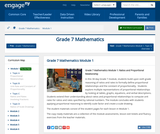Conditional Remix & Share Permitted
CC BY-NC-SA
Rating
0.0 stars

In this 30-day Grade 7 module, students build upon sixth grade reasoning of ratios and rates to formally define proportional relationships and the constant of proportionality.  Students explore multiple representations of proportional relationships by looking at tables, graphs, equations, and verbal descriptions.  Students extend their understanding about ratios and proportional relationships to compute unit rates for ratios and rates specified by rational numbers. The module concludes with students applying proportional reasoning to identify scale factor and create a scale drawing.

Subject:
Ratios and Proportions
Material Type:
Module
Provider:
New York State Education Department
Provider Set:
EngageNY
05/14/2013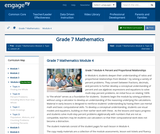Conditional Remix & Share Permitted
CC BY-NC-SA
Rating
0.0 stars

In Module 4, students deepen their understanding of ratios and proportional relationships from Module 1 by solving a variety of percent problems. They convert between fractions, decimals, and percents to further develop a conceptual understanding of percent and use algebraic expressions and equations to solve multi-step percent problems. An initial focus on relating 100% to the whole serves as a foundation for students.  Students begin the module by solving problems without using a calculator to develop an understanding of the reasoning underlying the calculations.  Material in early lessons is designed to reinforce students understanding by having them use mental math and basic computational skills. To develop a conceptual understanding, students use visual models and equations, building on their earlier work with these.  As the lessons and topics progress and students solve multi-step percent problems algebraically with numbers that are not as compatible, teachers may let students use calculators so that their computational work does not become a distraction.

Subject:
Ratios and Proportions
Material Type:
Module
Provider:
New York State Education Department
Provider Set:
EngageNY
01/02/2014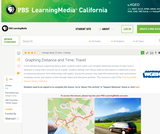Educational Use
Rating
0.0 stars

In this lesson designed to enhance literacy skills, students learn how to read and interpret a distance&ndash;time graph.

Subject:
Mathematics
Algebra
Physics
Material Type:
Activity/Lab
Provider:
PBS LearningMedia
Provider Set:
PBS Learning Media Common Core Collection
Author:
Walmart Foundation
WGBH Educational Foundation
08/21/2012Rating
4.0 stars

Use the Sound Grapher to create visualizations of sound and learn about the frequency, wavelength, amplitude and velocity of sound waves.

Subject:
Chemistry
Physics
Material Type:
Activity/Lab
Lecture Notes
Provider:
Concord Consortium
Provider Set:
Concord Consortium Collection
Author:
The Concord Consortium
12/11/2011Conditional Remix & Share Permitted
CC BY-NC
Rating
0.0 stars

In this problem-based learning module, students will be given the chance to plan their idea of the perfect party.  They are given a budget of $2,500, this is the maximum amount of money they can use. The goal is for students to plan a party that they think people would want to attend and would enjoy being a part of. The students will need to come up with categories of what their party will need (food/drink, decorations, entertainment, location, etc). These will then be the stations students will move at their own pace through to complete the party planning. At each station they will need to identify what they are doing to have/do for the party and how much it will cost. They will then have to figure out the unit cost (cost per person) for that category. The final station should allow for students to find the total cost of their part and total unit cost per person for the party. If the total cost exceeds$2,500 students should make adjustments as needed.Students will then create an advertisement (commercial, flyer, poster etc.) to promote their party as the “PARTY OF THE YEAR!”Students will then present these advertisements to school staff, parents, administrators etc. to vote on the party they would want to throw for their own child. They should take into consideration cost per person, entertainment, and enjoyment of the party.

Subject:
Mathematics
Material Type:
Lesson Plan
Author:
Blended Learning Teacher Practice Network
07/27/2018Only Sharing Permitted
CC BY-NC-ND
Rating
4.0 stars

This lesson unit is intended to help teachers assess how well students are able to interpret percent increase and decrease, and in particular, to identify and help students who have the following difficulties: translating between percents, decimals, and fractions; representing percent increase and decrease as multiplication; and recognizing the relationship between increases and decreases.

Subject:
Ratios and Proportions
Material Type:
Assessment
Lesson Plan
Provider:
Shell Center for Mathematical Education
Provider Set:
Mathematics Assessment Project (MAP)
04/26/2013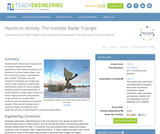Educational Use
Rating
0.0 stars

Students learn about radar imaging and its various military and civilian applications that include recognition and detection of human-made targets, and the monitoring of space, deforestation and oil spills. They learn how the concepts of similarity and scaling are used in radar imaging to create three-dimensional models of various targets. Students apply the critical attributes of similar figures to create scale models of a radar imaging scenario using infrared range sensors (to emulate radar functions) and toy airplanes (to emulate targets). They use technology tools to measure angles and distances, and relate the concept of similar figures to real-world applications.

Subject:
Education
Material Type:
Activity/Lab
Provider:
TeachEngineering
Provider Set:
TeachEngineering
Author:
Mounir Ben Ghalia
Rocio Denise Nava
10/14/2015Conditional Remix & Share Permitted
CC BY-NC
Rating
0.0 stars

Four full-year digital course, built from the ground up and fully-aligned to the Common Core State Standards, for 7th grade Mathematics. Created using research-based approaches to teaching and learning, the Open Access Common Core Course for Mathematics is designed with student-centered learning in mind, including activities for students to develop valuable 21st century skills and academic mindset.

Subject:
Mathematics
Material Type:
Full Course
Provider:
Pearson
10/06/2016Rating
0.0 stars

Getting Started

Type of Unit: Introduction

Prior Knowledge

Students should be able to:

Understand ratio concepts and use ratios.
Use ratio and rate reasoning to solve real-world problems.
Identify and use the multiplication property of equality.

Lesson Flow

This unit introduces students to the routines that build a successful classroom math community, and it introduces the basic features of the digital course that students will use throughout the year.

An introductory card sort activity matches students with their partner for the week. Then over the course of the week, students learn about the routines of Opening, Work Time, Ways of Thinking, Apply the Learning (some lessons), Summary of the Math, Reflection, and Exercises. Students learn how to present their work to the class, the importance of students’ taking responsibility for their own learning, and how to effectively participate in the classroom math community.

Students then work on Gallery problems, to further explore the resources and tools and to learn how to organize their work.

The mathematical work of the unit focuses on ratios and rates, including card sort activities in which students identify equivalent ratios and match different representations of an equivalent ratio. Students use the multiplication property of equality to justify solutions to real-world ratio problems.

Subject:
Mathematics
Material Type:
Unit of Study
Provider:
PearsonConditional Remix & Share Permitted
CC BY-NC
Rating
0.0 stars

Discuss the important ways that listeners contribute to mathematical discussions during Ways of Thinking presentations. Then use ratio and rate reasoning to solve a problem about ingredients in a stew.Key ConceptsStudents find the unit rate of a ratio situation.Goals and Learning ObjectivesContribute as listeners during the Ways of Thinking discussion.Understand the concept of a unit rate that is associated with a ratio.Use rate reasoning to solve real-world problems.

Subject:
Ratios and Proportions
Material Type:
Lesson Plan
Provider:
Pearson
09/21/2015Rating
0.0 stars

Proportional Relationships

Type of Unit: Concept

Prior Knowledge

Students should be able to:

Understand what a rate and ratio are.
Make a ratio table.
Make a graph using values from a ratio table.

Lesson Flow

Students start the unit by predicting what will happen in certain situations. They intuitively discover they can predict the situations that are proportional and might have a hard time predicting the ones that are not. In Lessons 2–4, students use the same three situations to explore proportional relationships. Two of the relationships are proportional and one is not. They look at these situations in tables, equations, and graphs. After Lesson 4, students realize a proportional relationship is represented on a graph as a straight line that passes through the origin. In Lesson 5, they look at straight lines that do not represent a proportional relationship. Lesson 6 focuses on the idea of how a proportion that they solved in sixth grade relates to a proportional relationship. They follow that by looking at rates expressed as fractions, finding the unit rate (the constant of proportionality), and then using the constant of proportionality to solve a problem. In Lesson 8, students fine-tune their definition of proportional relationship by looking at situations and determining if they represent proportional relationships and justifying their reasoning. They then apply what they have learned to a situation about flags and stars and extend that thinking to comparing two prices—examining the equations and the graphs. The Putting It Together lesson has them solve two problems and then critique other student work.

Gallery 1 provides students with additional proportional relationship problems.

The second part of the unit works with percents. First, percents are tied to proportional relationships, and then students examine percent situations as formulas, graphs, and tables. They then move to a new context—salary increase—and see the similarities with sales taxes. Next, students explore percent decrease, and then they analyze inaccurate statements involving percents, explaining why the statements are incorrect. Students end this sequence of lessons with a formative assessment that focuses on percent increase and percent decrease and ties it to decimals.

Students have ample opportunities to check, deepen, and apply their understanding of proportional relationships, including percents, with the selection of problems in Gallery 2.

Subject:
Mathematics
Ratios and Proportions
Material Type:
Unit of Study
Provider:
PearsonConditional Remix & Share Permitted
CC BY-NC
Rating
0.0 stars

Students analyze the graph of a proportional relationship in order to find the approximate constant of proportionality, to write the related formula, and to create a table of values that lie on the graph.Key ConceptsThe constant of proportionality determines the steepness of the straight-line graph that represents a proportional relationship. The steeper the line is, the greater the constant of proportionality.On the graph of a proportional relationship, the constant of proportionality is the constant ratio of y to x, or the slope of the line.A proportional relationship can be represented in different ways: a ratio table, a graph of a straight line through the origin, or an equation of the form y = kx, where k is the constant of proportionality.Goals and Learning ObjectivesIdentify the constant of proportionality from a graph that represents a proportional relationship.Write a formula for a graph that represents a proportional relationship.Make a table for a graph that represents a proportional relationship.Relate the constant of proportionality to the steepness of a graph that represents a proportional relationship (i.e., the steeper the line is, the greater the constant of proportionality).

Subject:
Ratios and Proportions
Material Type:
Lesson Plan
Provider:
Pearson
09/21/2015Conditional Remix & Share Permitted
CC BY-NC
Rating
0.0 stars

Students connect percent to proportional relationships in the context of sales tax.Key ConceptsWhen there is a constant tax percent, the total cost for items purchase—including the price and the tax—is proportional to the price.To find the cost, c , multiply the price of the item, p, by (1 + t), where t is the tax percent, written as a decimal: c = p(1 + t).The constant of proportionality is (1 + t) because of the structure of the situation:c = p + tp = p(1 + t).Because of the distributive property, multiplying the price by (1 + t) means multiplying the price by 1, then multiplying the price by t, and then taking the sum of these products.Goals and Learning ObjectivesFind the total cost in a sales tax situation.Understand that a proportional relationship only exists between the price of an item and the total cost of the item if the sales tax is constant.Find the constant of proportionality in a sales tax situation.Make a graph of an equation showing the relationship between the price of an item and the total amount paid.

Subject:
Ratios and Proportions
Material Type:
Lesson Plan
Provider:
Pearson
09/21/2015Conditional Remix & Share Permitted
CC BY-NC
Rating
0.0 stars

Lesson OverviewStudents calculate the constant of proportionality for a proportional relationship based on a table of values and use it to write a formula that represents the proportional relationship.Key ConceptsIf two quantities are proportional to one another, the relationship between them can be defined by a formula of the form y = kx, where k is the constant ratio of y-values to corresponding x-values. The same relationship can also be defined by the formula x=(1k)y , where 1k is now the constant ratio of x-values to y-values.Goals and Learning ObjectivesDefine the constant of proportionality.Calculate the constant of proportionality from a table of values.Write a formula using the constant of proportionality.

Subject:
Ratios and Proportions
Material Type:
Lesson Plan
Provider:
Pearson
09/21/2015Conditional Remix & Share Permitted
CC BY-NC
Rating
0.0 stars

Students write the relationship between two fractions as a unit rate and use unit rates and the constant of proportionality to solve problems involving proportional relationships.Key ConceptsIn situations where there is a constant rate involved, the unit rate is a constant of proportionality between the two variable quantities and can be used to write a formula of the form y = kx.A given constant rate can be simplified to find the unit rate by expressing its value with a denominator of 1.The ratios of two fractions can be expressed as a unit rate.Goals and Learning ObjectivesExpress the ratios of two fractions as a unit rate.Understand that when a constant rate is involved, the unit rate is the constant of proportionality.Use the unit rate to write and solve a formula of the form y = kx.

Subject:
Ratios and Proportions
Material Type:
Lesson Plan
Provider:
Pearson
09/21/2015Conditional Remix & Share Permitted
CC BY-NC
Rating
0.0 stars

Students look at the relationship between the number of flags manufactured and the stars on the flag and determine whether it represents a proportional relationship.Key ConceptsThe form of the equation of a proportional relation is y = kx, where k is the constant of proportionality.A graph of a proportional relationship is a straight line that passes through the origin.The constant of a proportionality in a graph of a proportional relationship is the constant ratio of y to x (the slope of the line).Goals and Learning ObjectivesIdentify the constant of proportionality in a proportional relationship based on a real-world problem situation.Write a formula using the constant of proportionality.Analyze a graph of a proportional relationship.Make a graph and determine if it represents a proportional relationship.Identify the constant of proportionality in a graph of a proportional relationship.

Subject:
Ratios and Proportions
Material Type:
Lesson Plan
Provider:
Pearson
09/21/2015Conditional Remix & Share Permitted
CC BY-NC
Rating
0.0 stars

Students explore the idea that not all straight lines are proportional by comparing a graph representing a stack of books with a graph representing a stack of cups. They recognize that all proportional relationships are represented as a straight line that passes through the origin.Key ConceptsNot all graphs of straight lines represent proportional relationships.There are three ways to tell whether a relationship between two varying quantities is proportional:The graph of the relationship between the quantities is a straight line that passes through the point (0, 0).You can express one quantity in terms of the other using a formula of the form y = kx.The ratios between the varying quantities are constant.Goals and Learning ObjectivesUnderstand when a graph of a straight line is and when it is not a proportional relationship.Recognize that a proportional relationship is shown on a graph as a straight line that passes through the origin (0, 0).Make a table of values to represent two quantities that vary.Graph a table of values representing two quantities that vary.Describe what each variable and number in a formula represents.

Subject:
Ratios and Proportions
Material Type:
Lesson Plan
Provider:
Pearson
09/21/2015Conditional Remix & Share Permitted
CC BY-NC
Rating
0.0 stars

Students continue to explore the three relationships from the previous lessons: Comparing Dimensions, Driving to the Amusement Park, and Temperatures at the Amusement Park. They graph the three situations and realize that the two proportional relationships form a straight line, but the time and temperature relationship does not.Key ConceptsA table of values that represent equivalent ratios can be graphed in the coordinate plane. The graph represents a proportional relationship in the form of a straight line that passes through the origin (0, 0). The unit rate is the slope of the line.Goals and Learning ObjectivesRepresent relationships shown in a table of values as a graph.Recognize that a proportional relationship is shown on a graph as a straight line that passes through the origin (0, 0).

Subject:
Ratios and Proportions
Material Type:
Lesson Plan
Provider:
Pearson
09/21/2015Conditional Remix & Share Permitted
CC BY-NC
Rating
0.0 stars

Students have an opportunity to review their own work on the Self Check in the previous lesson, consider feedback that addresses specific aspects of their work, examine a different approach to the problem from the Self Check, and then use what they learned to solve a closely related problem.Key ConceptsStudents reflect on their work, review and critique student work on the same problem, and then apply their learning to solve a similar problem.Goals and Learning ObjectivesUse teacher comments to refine their solution strategies for a proportional relationship problemDeepen their understanding of proportional relationships.Synthesize and connect strategies for representing and investigating proportional relationships.Critique given student work involving proportional relationships.Apply deepened understanding of proportional relationships to a new problem situation.

Subject:
Mathematics
Material Type:
Lesson Plan
Provider:
Pearson
09/21/2015Conditional Remix & Share Permitted
CC BY-NC
Rating
0.0 stars

Students watch a video showing three different ways to solve a problem involving a proportional relationship, and then they use each method to solve a similar problem. Students describe each approach, including the mathematical terms associated with each.Key ConceptsThree methods for solving problems involving proportional relationships include:Setting up a proportion and solving for the missing valueFinding the unit rate and multiplyingWriting and solving a formula using the constant of proportionalityGoals and Learning ObjectivesSolve a problem involving a proportional relationship in three different ways: set up a proportion and solve for a missing value, use a unit rate, and use the constant of proportionality to write and solve a formula.

Subject:
Ratios and Proportions
Material Type:
Lesson Plan
Provider:
Pearson
09/21/2015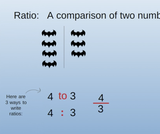Unrestricted Use
CC BY
Rating
5.0 stars

This lesson teaches about Rates, Ratios, and Unit Rates, using visually appealing art and real-life examples that will engage teenagers.

Subject:
Ratios and Proportions
Material Type:
Lesson
Author:
Janel Williams
01/15/2021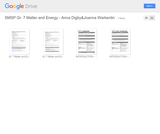Conditional Remix & Share Permitted
CC BY-NC
Rating
0.0 stars

Using the mission to land a human on the Martian surface as context, students will use knowledge about energy and molecular motion to build and test a simplified heat shield.

Subject:
Physical Science
Physics
Material Type:
Activity/Lab
Lesson Plan
Provider:
South Metro-Salem STEM Partnership
Author:
Anna Digby & Joanna Warkentin
07/01/2015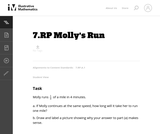Unrestricted Use
CC BY
Rating
0.0 stars

This task asks students to solve a problem in a context involving constant speed. This task provides a transition from working with ratios involving whole numbers to ratios involving fractions.

Subject:
Mathematics
Material Type:
Activity/Lab
Provider:
Illustrative Mathematics
Provider Set:
Illustrative Mathematics
Author:
Illustrative Mathematics